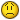# database designing for paying monthly salary

G

#### Guest

I'm designing a monthly salary database in ms access and VBA that has the
following tables:
1- personal table:
fields:id,name,family,disabled(0=not disabled,1=50% disabled, 2=100%
disabled),insurancetype(1=7%,2=9%)
2- monetary table:
fields:id,salary,overtimehour,lunchmoney,tax
3-debt table:
fields:id,remainder,payment
4-year table:
field:year
5-month table:
field:month
how can I create a year that each of twelve months have 30 days since a year
has 360 days?

1-the tax list is so:
if sum of salary,overtime and lunchmoneny <= 1350000 then tax rate=0%
if sum of salary,overtime and lunchmoneny > 1350000 then tax rate=10%
if value of disabled is equal to 0 then tax is calculated as above
if value of disabled is equal to 1 then tax is calculated as above
multiplied by 50%
if value of disabled is equal to 2 then tax is 0 for that record
2-insurance:
if value of insurancetype is 1 the insurance is calculated
sosalary+overtimehour)*7%
if value of insurancetype is 2 the insurance is calculated sosalary)*9%
3-overtimehour:
the formula for overtime is so: overtime=(salary/160)*overtimehour
4-lunchmoney:
the value of this field for each month is fix. For example a person gets
200000 and another person doesn't get anything.
5-remainder,payment:
for example if a person in month 4 borrows 500000 with monthly payment
100000 we have the following list:
month remainder payment
4 500000 100000
5 400000 100000
6 300000 100000
7 200000 100000
8 100000 100000
9 0 0
in addition if this person in month 6 borrows 200000 and monthly payment
100000 we have the following list:
month remainder
payment
4 500000
100000
5 400000
100000
6 500000(=300000+200000)
200000(=100000+100000)
7 300000
200000(=100000+100000)
8 100000
100000
9 0
if this person in month 6 settles previous loan and borrows a new loanfor
example 900000 and monthly payment 150000, we have the following list:
month remainder
payment
4 500000
100000
5 400000
100000
6 900000(=0+900000)
150000(=0+150000)
7 750000
150000
8 600000
150000
9 450000
150000
10 300000
150000
11 150000
150000
12 0

if a person borrows 600000 in month 11 with monthly payment 250000 , we have
the following list:
month remainder
payment
11 600000
250000
12 350000
250000
1(next year) 100000
250000
2 0
how can we automatically transfer values of these two fields to the next
year as above?
In month 1 of next year the value of remainder field is less than the value
of payment, then how can we replace the value of payment(250000) with the
value of remainder(100000) as below:
month remainder
payment
11 600000
250000
12 350000
250000
1(next year) 100000
100000
2 0

questions:
1-can I make a unique form that entry data to personal , monetary and debt
tables ?

2-if a person gets 200000 lunch money for 30 days but this value increases
to 250000 at 21-6-1984 how can I calculate difference 50000 for 10/30 days of
month 6 and show it separately?in month 7 and after that this value will be
250000.

3-how can I create new month? For example we have calculated salary of month
6 and we want to create month 7 that salary and lunchmoney are fix can come
to records of month 7 but overtimehour that is not fix we entry data for it.

4-how can I create new year?
5-how can I calculate some field every two months?for example if lunchmoney
is 200000 for each month and I donâ€™t calculate it for this month I must
calculate it in the next month double for example 200000*2.

6-how can I prevent to calculate the salary of a person that to be retired
for next months?

7-how can I prevent to calculate the salary of a person for example only
this month but calculate it for next month?

Sincerely yours
amini

J

#### Jamie Collins

aminihojat said:
I'm designing a monthly salary database

I *think* the majority of your questions can be answered by taking a
different approach to modelling time.

Take, for example, your question, "how can I prevent to calculate the
salary of a person that to be retired for next months?"

To answer this one, you could have a start_date and end_dates for the
employee; more practical may be to model periods of salary history (see
my example here:

To support this, you could use a Calendar auxiliary table. This would
have one row for each considered day in your enterprise (e.g. 30 day
months), with columns for reporting year, month number, month start
date, month end date, etc. Yes, storing data in this way is redundant
but if it makes your life easier then why not? This is an auxiliary
table and a standard trick, after all.

So your query to determine who will be paid this month will be more a
case of determining how employee's employment/earnings dates overlap
the considered dates of the current enterprise month.

I hope this gives you some ideas.

Jamie.

--